# Search by Topic

#### Resources tagged with Area - triangles, quadrilaterals, compound shapes similar to Triangle Transformation:

Filter by: Content type:
Age range:
Challenge level:

### There are 20 results

Broad Topics > Measuring and calculating with units > Area - triangles, quadrilaterals, compound shapes### Triangle Transformation

##### Age 7 to 14 Challenge Level:

Start with a triangle. Can you cut it up to make a rectangle?### Kissing Triangles

##### Age 11 to 14 Challenge Level:

Determine the total shaded area of the 'kissing triangles'.### Isosceles

##### Age 11 to 14 Challenge Level:

Prove that a triangle with sides of length 5, 5 and 6 has the same area as a triangle with sides of length 5, 5 and 8. Find other pairs of non-congruent isosceles triangles which have equal areas.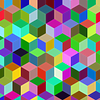### More Isometric Areas

##### Age 11 to 14 Challenge Level:

Isometric Areas explored areas of parallelograms in triangular units. Here we explore areas of triangles...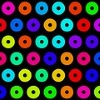### Isometric Areas

##### Age 11 to 14 Challenge Level:

We usually use squares to measure area, but what if we use triangles instead?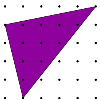### Triangles in a Square

##### Age 11 to 14 Challenge Level:

What are the possible areas of triangles drawn in a square?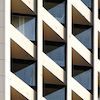### Completing Quadrilaterals

##### Age 11 to 14 Challenge Level:

We started drawing some quadrilaterals - can you complete them?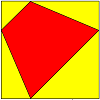### Quadrilaterals in a Square

##### Age 11 to 14 Challenge Level:

What's special about the area of quadrilaterals drawn in a square?### Dotty Triangles

##### Age 11 to 14 Challenge Level:

Imagine an infinitely large sheet of square dotty paper on which you can draw triangles of any size you wish (providing each vertex is on a dot). What areas is it/is it not possible to draw?### Tilted Squares

##### Age 11 to 14 Challenge Level:

It's easy to work out the areas of most squares that we meet, but what if they were tilted?### Overlap

##### Age 11 to 14 Challenge Level:

A red square and a blue square overlap so that the corner of the red square rests on the centre of the blue square. Show that, whatever the orientation of the red square, it covers a quarter of the. . . .### Disappearing Square

##### Age 11 to 14 Challenge Level:

Do you know how to find the area of a triangle? You can count the squares. What happens if we turn the triangle on end? Press the button and see. Try counting the number of units in the triangle now. . . .### Linkage

##### Age 11 to 14 Challenge Level:

Four rods, two of length a and two of length b, are linked to form a kite. The linkage is moveable so that the angles change. What is the maximum area of the kite?### Shear Magic

##### Age 11 to 14 Challenge Level:

What are the areas of these triangles? What do you notice? Can you generalise to other "families" of triangles?### Rati-o

##### Age 11 to 14 Challenge Level:

Points P, Q, R and S each divide the sides AB, BC, CD and DA respectively in the ratio of 2 : 1. Join the points. What is the area of the parallelogram PQRS in relation to the original rectangle?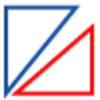### Uncanny Triangles

##### Age 7 to 11 Challenge Level:

Can you help the children find the two triangles which have the lengths of two sides numerically equal to their areas?### Isosceles Triangles

##### Age 11 to 14 Challenge Level:

Draw some isosceles triangles with an area of $9$cm$^2$ and a vertex at (20,20). If all the vertices must have whole number coordinates, how many is it possible to draw?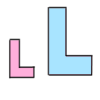### Growing Rectangles

##### Age 11 to 14 Challenge Level:

What happens to the area and volume of 2D and 3D shapes when you enlarge them?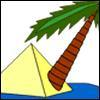### Triangle Island

##### Age 7 to 11 Challenge Level:

You have pitched your tent (the red triangle) on an island. Can you move it to the position shown by the purple triangle making sure you obey the rules?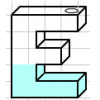### Maths Filler

##### Age 11 to 14 Challenge Level:

Imagine different shaped vessels being filled. Can you work out what the graphs of the water level should look like?